#### Thank you for registering.

One of our academic counsellors will contact you within 1 working day.

Please check your email for login details.

Click to Chat

1800-1023-196

+91-120-4616500

CART 0

• 0
MY CART (5)

Use Coupon: CART20 and get 20% off on all online Study Material

ITEM
DETAILS
MRP
DISCOUNT
FINAL PRICE
Total Price: Rs.

There are no items in this cart.
Continue Shopping• Complete JEE Main/Advanced Course and Test Series
• Offered Price: Rs. 15,900
• View Details

# Chapter 4: Triangles Exercise – 4.3

### Question: 1

In a Δ ABC, AD is the bisector of ∠A, meeting side BC at D.

(i) If BD = 2.5 cm, AB = 5 cm, and AC = 4.2 cm, find DC.

(ii) If BD = 2 cm, AB = 5 cm, and DC = 3 cm, find AC.

(iii)  If AB = 3.5 cm, AC = 4.2 cm, and DC = 2.8 cm, find BD.

(iv)  If AB = 10 cm, AC = 14 cm, and BC = 6 cm, find BD and DC.

(v) If AC = 4.2 cm, DC = 6 cm, and BC = 10 cm, find AB.

(vi) If AB = 5.6 cm, BC = 6 cm, and DC = 3 cm, find BC.

(vii) If AB = 5.6 cm, BC = 6 cm, and BD = 3.2 cm, find AC.

(viii) If AB = 10 cm, AC = 6 cm, and BC = 12 cm, find BD and DC.

### Solution:

(i) It is given that BD = 2.5 cm, AB = 5 cm, and AC = 4.2 cm.

In Δ ABC, AD is the bisector of ∠A, meeting side BC at D.

We need to find DC,

Since, AD is ∠A bisector,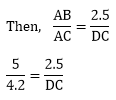5DC = 4.2 x 2.5

DC = (4.2 x 2.5)/5

DC = 2.1

(ii) It is given that BD = 2 cm, AB = 5 cm, and DC = 3 cm

In Δ ABC, AD is the bisector of ∠A, meeting side BC at D

We need to find AC.

Since, AD is ∠A bisector.

Therefore,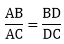(since AD is the bisector of ∠A and side BC)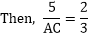2AC = 5 × 3

AC = 15/2

AC = 7.5 cm

(iii) It is given that AB = 3.5 cm, AC = 4.2 cm, and DC = 2.8 cm

In Δ ABC, AD is the bisector of ∠A, meeting side BC at D

We need to find BD.

Since, AD is ∠A bisector

Therefore,(since, AD is the bisector of ∠A and side  BC)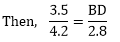BD = (3.5 × 2.8)/4.2

BD = 7/3

BD = 2.3 cm

(iv) It is given that AB = 10 cm, AC = 14 cm, and BC = 6 cm

In Δ ABC, AD is the bisector of ∠A meeting side BC at D

We need to find BD and DC.

Since, AD is bisector of ∠A

Therefore,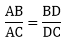(AD is bisector of ∠ A and side BC)14x = 60 – 6x

20x = 60

x = 60/20

BD = 3 cm and DC = 3 cm.

(v) It is given that AC = 4.2 cm, DC = 6 cm, and BC = 10 cm.

In Δ ABC, AD is the bisector of ∠A, meeting side BC at D.

We need to find out AB,

Since, AD is the bisector of ∠A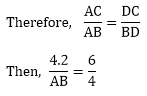6AB = 4.2 x 4

AB = (4.2 x 4)/6

AB = 16.8/6

AB = 2.8 cm

(vi) It is given that AB = 5.6 cm, BC = 6 cm, and DC = 3 cm

In Δ ABC,  AD is the bisector of  ∠A,  meeting side BC at D

We need to find BC,

Since, AD is the ∠A bisector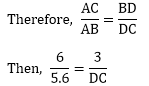DC = 2.8 cm

And, BC = 2.8 + 3

BC = 5.8 cm

(vii) It is given that AB = 5.6 cm, BC = 6 cm, and BD = 3.2 cm

In Δ ABC,  AD is the bisector of ∠A , meeting side BC at D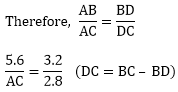AC = (5.6 x 2.8)/3.2

AC = 4.9 cm

(viii) It is given that AB = 10 cm, AC = 6 cm, and BC = 12 cm

In Δ ABC, AD is the ∠A bisector, meeting side BC at D.

We need to find BD and DC

Since, AD is bisector of ∠A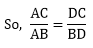Let BD = x cm

Then,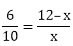6x = 120 – 10x

16x = 120

x = 120/16

x = 7.5

Now, DC = 12 – BD

DC = 12 – 7.5

DC = 4.5

BD = 7.5 cm and DC = 4.5 cm.

### Question: 2

AE is the bisector of the exterior ∠CAD meeting BC produced in E. If AB = 10 cm, AC = 6 cm, and BC = 12 cm, Find CE.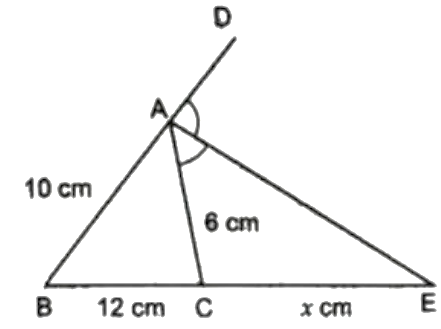### Solution:

It is given that AE is the bisector of the exterior ∠CAD

Meeting BC produced E and AB = 10 cm, AC = 6 cm, and BC = 12 cm.

Since AE is the bisector of the exterior ∠CAD.72 + 6x = 10x

4x = 72

x = 18

CE = 18 cm

### Question: 3

Δ ABC is a triangle such that AB/AC = BD/DC, ∠B = 70, ∠C = 50, find ∠BAD.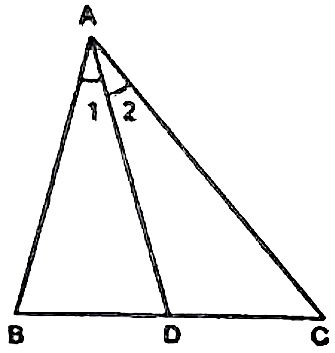### Solution:

It is given that in Δ ABC, ABAC = BDDC AB/AC = BD/DC, ∠B = 70 and ∠C = 50

We need to find ∠BAD

In Δ ABC,

∠A = 180 - (70 + 50)

= 180 - 120

= 60

Since, AB/AC = BD/DC

Therefore, AD is the bisector of ∠A

Hence, ∠BAD = 60/2 = 30

### Question: 4

Check whether AD is the bisector of ∠A of ΔABC in each of the following: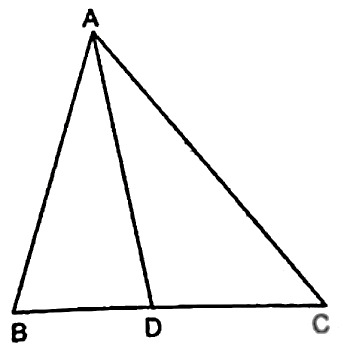(i) AB = 5 cm, AC = 10 cm, BD = 1.5 cm and CD = 3.5 cm

(ii) AB = 4 cm, AC = 6 cm, BD = 1.6 cm and CD = 2.4 cm

(iii)  AB = 8 cm, AC = 24 cm, BD = 6 cm and BC = 24 cm

(iv)  AB = 6 cm, AC = 8 cm, BD = 1.5 cm and CD = 2 cm

(v)  AB = 5 cm, AC = 12 cm, BD = 2.5 cm and BC = 9 cm

### Solution:

(i) It is given that AB = 5 cm, AC = 10 cm, BD = 1.5 cm and CD = 3.5 cm

We have to check whether AD is bisector of ∠A

First we will check proportional ratio between sides.

Now,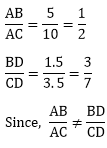Hence, AD is not the bisector of ∠A.

(ii) It is given that AB = 4 cm, AC = 6 cm, BD = 1.6 cm and CD = 2.4 cm.

We have to check whether AD is the bisector of ∠A

First we will check proportional ratio between sides.Hence, AD is the bisector of ∠A.

(iii) It is given that AB = 8 cm, AC = 24 cm, BD = 6 cm and BC = 24 cm.

We have to check whether AD is the bisector of ∠A

First we will check proportional ratio between sides.

DC = BC – BD

DC = 24 – 6

DC = 18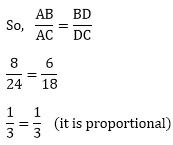(iv) It is given that AB = 6 cm, AC = 8 cm, BD = 1.5 cm and CD = 2 cm.

We have to check whether AD is the bisector of ∠A

First, we will check proportional ratio between sides.Hence, AD is the bisector of ∠A.

(v) It is given that AB = 5 cm, AC = 12 cm, BD = 2.5 cm and BC = 9 cm.

We have to check whether AD is the bisector of ∠A

First, we will check proportional ratio between sides.Hence, AD is not the bisector of ∠A.

### Question: 5

In fig. AD bisects ∠A, AB = 12 cm, AC = 20 cm, and BD = 5 cm, determine CD.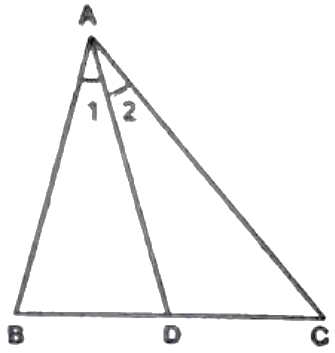### Solution:

It is given that AD bisects ∠A

AB = 12 cm, AC = 20 cm, and BD = 5 cm.

We need to find CD.

Since AD is the bisector of ∠A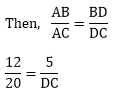12 × DC = 20 × 5

DC = 100/12

DC = 8.33 cm

∴  CD = 8.33 cm.

### Question: 6

In ΔABC, if ∠1 = ∠2, prove that,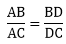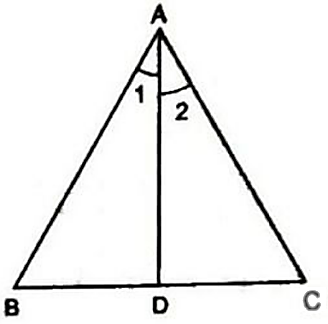### Solution:

We need to prove that,In  ΔABC,

∠1 = ∠2

So, AD is the bisector of ∠A

Therefore,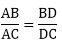### Question: 7

D and E are the points on sides BC, CA and AB respectively. of a ΔABC  such that AD bisects  ∠A, BE bisects ∠B and CF bisects ∠C. If AB = 5 cm, BC = 8 cm, and CA = 4 cm, determine AF, CE, and BD.

### Solution:

It is given that AB = 5 cm, BC = 8 cm and CA = 4 cm.

We need to find out, AF, CE and BD.

Since, AD is the bisector of ∠A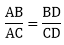Then,40 – 5B D = 4 BD

9 BD = 40

So, BD = 40/9

Since, BE is the bisector of ∠B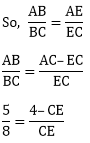5CE = 32 – 8CE

5CE + 8CE = 32

13CE = 32

So, CE = 32/13

Now, since, CF is the bisector of ∠C8AF = 20 – 4AF

12AF = 20

So, 3AF = 5

AF = 5/3 cm, CE = 32/12 cm

and BD = 40/9 cm## Complete JEE Main/Advanced Course and Test Series

OFFERED PRICE: Rs. 15,900
View Details Xpress Buy

### Course Features

• 731 Video Lectures
• Revision Notes
• Test paper with Video Solution
• Mind Map
• Study Planner
• NCERT Solutions
• Discussion Forum
• Previous Year Papers

### Course Features

• 728 Video Lectures
• Revision Notes
• Previous Year Papers
• Mind Map
• Study Planner
• NCERT Solutions
• Discussion Forum
• Test paper with Video Solution